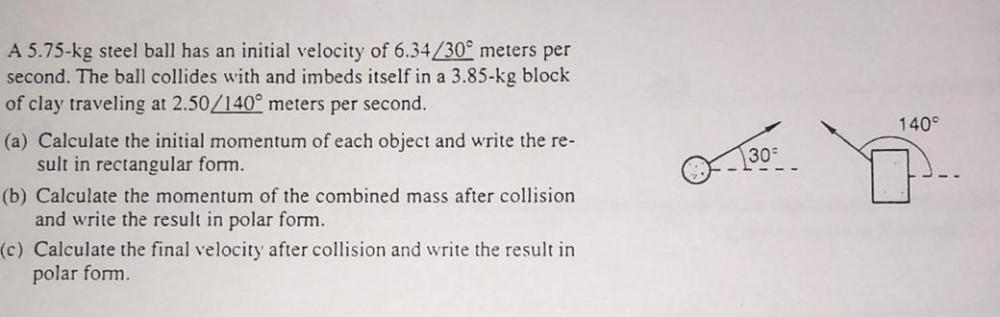Question:

# 140 A 5.75-kg steel ball has an initial velocity of 6.34/30 meters per second. The ball collides with and imbeds itself in a 3.8140 A 5.75-kg steel ball has an initial velocity of 6.34/30 meters per second. The ball collides with and imbeds itself in a 3.85-kg block of clay traveling at 2.50/140° meters per second. (a) Calculate the initial momentum of each object and write the re- sult in rectangular form. (b) Calculate the momentum of the combined mass after collision and write the result in polar form. (c) Calculate the final velocity after collision and write the result in polar form 300